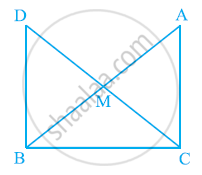# In right triangle ABC, right angled at C, M is the mid-point of hypotenuse AB. C is joined to M and produced to a point D such that DM = CM. - Mathematics

In right triangle ABC, right angled at C, M is the mid-point of hypotenuse AB. C is joined to M and produced to a point D such that DM = CM. Point D is joined to point B (see the given figure). Show that:

(i) ΔAMC ≅ ΔBMD

(ii) ∠DBC is a right angle.

(iii) ΔDBC ≅ ΔACB

(iv) CM = 1/2AB#### Solution

(i) In ΔAMC and ΔBMD,

AM = BM (M is the mid-point of AB)

∠AMC = ∠BMD (Vertically opposite angles)

CM = DM (Given)

∴ ΔAMC ≅ ΔBMD (By SAS congruence rule)

∴ AC = BD (By CPCT)

And, ∠ACM = ∠BDM (By CPCT)

(ii) ∠ACM = ∠BDM

However, ∠ACM and ∠BDM are alternate interior angles.

Since alternate angles are equal,

It can be said that DB || AC

⇒ ∠DBC + ∠ACB = 180º (Co-interior angles)

⇒ ∠DBC + 90º = 180º

⇒ ∠DBC = 90º

(iii) In ΔDBC and ΔACB,

∠DBC = ∠ACB (Each 90°)

BC = CB (Common)

∴ ΔDBC ≅ ΔACB (SAS congruence rule)

(iv) ΔDBC ≅ ΔACB

∴ AB = DC (By CPCT)

⇒ AB = 2 CM

∴ CM = 1/2 AB

Concept: Criteria for Congruence of Triangles
Is there an error in this question or solution?
Chapter 7: Triangles - Exercise 7.1 [Page 120]

#### APPEARS IN

NCERT Class 9 Maths
Chapter 7 Triangles
Exercise 7.1 | Q 8 | Page 120
Share